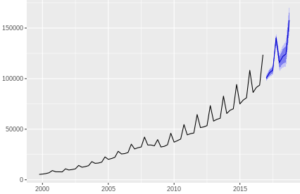Want to share your content on R-bloggers? click here if you have a blog, or here if you don't.There are two main approaches to time series forecasting. One of them is to find persistent patterns in a time series itself, and extrapolate those patterns. Another approach is to discover how a series depend on other variables, which serve as predictors.
This set of exercises focuses on the first approach, while the second one will be considered in a later set. The present set allows to practice in applying three forecasting models:
– a naive model, which provides probably the simplest forecasting technique, but still can be useful as a benchmark for evaluating other methods,
– a linear trend model (based on a simple linear regression),
– the ARIMA model, a more sophisticated and popular model, which assumes a linear dependence of a time series on its past values and random shocks.
The exercises do not require a deep understanding of underlying theories, and make use of automatic model estimation functions included in the `forecast` package. The set also help to practice in retrieving useful data from forecasts (confidence intervals, forecast errors), and comparing predictive accuracy of different models. The exercises are based on data on e-commerce retail sales in the USA for 1999-2016 retrieved from FRED, the Federal Reserve Bank of St. Louis database (download here). The data represent quarterly sales volumes in millions of dollars.

For other parts of the series follow the tag forecasting

Answers to the exercises are available here

Exercise 1
Read the data from the file, and transform it into a time series (`ts`) object (given that the data is quarterly and the starting period is the fourth quarter of 1999).
Plot the obtained series.

Exercise 2
Make a naive forecast for the next 8 periods using the appropriate function from the `forecast` package (i.e. create an object of the class `forecast` using the function that implements the naive method of forecasting) (Note that this method sets all forecast values equal to the last known time series value).

Exercise 3
Plot the forecast values.

Exercise 4
Make a forecast for the next 8 periods based on a linear model in two steps:
(1) create a linear regression model for the forecast using the `tslm` function from the `forecast` package (use the series as the dependent variable, trend and season as independent variables),
(2) make a forecast based on the model using the `forecast` function from the same package.
Plot the forecast.

Exercise 5
Retrieve forecast errors (residuals) from the linear model based forecast and save them as a separate variable.

Learn more about Forecasting in the online course Time Series Analysis and Forecasting in R. In this course you will learn how to:

• A complete introduction on Forecasting
• Work thru an exponentional smoothing instruction
• And much more

Exercise 6
Make a forecast for the next 8 periods based on the ARIMA model in two steps:
(1) create a model using the `auto.arima` function from the `forecast` package,
(2) make a forecast based on the model using the `forecast` function from the same package.
Plot the forecast.

Exercise 7
Print the summary of the forecast based on the ARIMA model.

Exercise 8
Explore the structure the forecast summary. Find the forecast value for the last period, and its 5% confidence interval values.

Exercise 9
Retrieve forecast errors (residuals) from the ARIMA based forecast.

Exercise 10
Use the errors from the ARIMA based forecast and the errors from the linear model based forecast to compare predictive accuracy of the two models with the Diebold-Mariano test (implemented as a function in the `forecast` package). Test the hypothesis that the ARIMA based forecast is more accurate than the linear model based forecast.